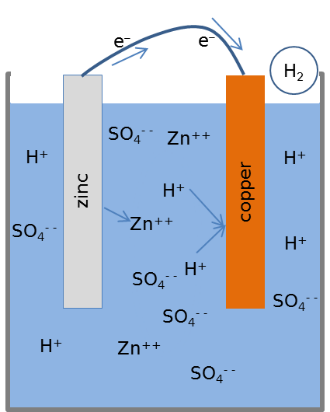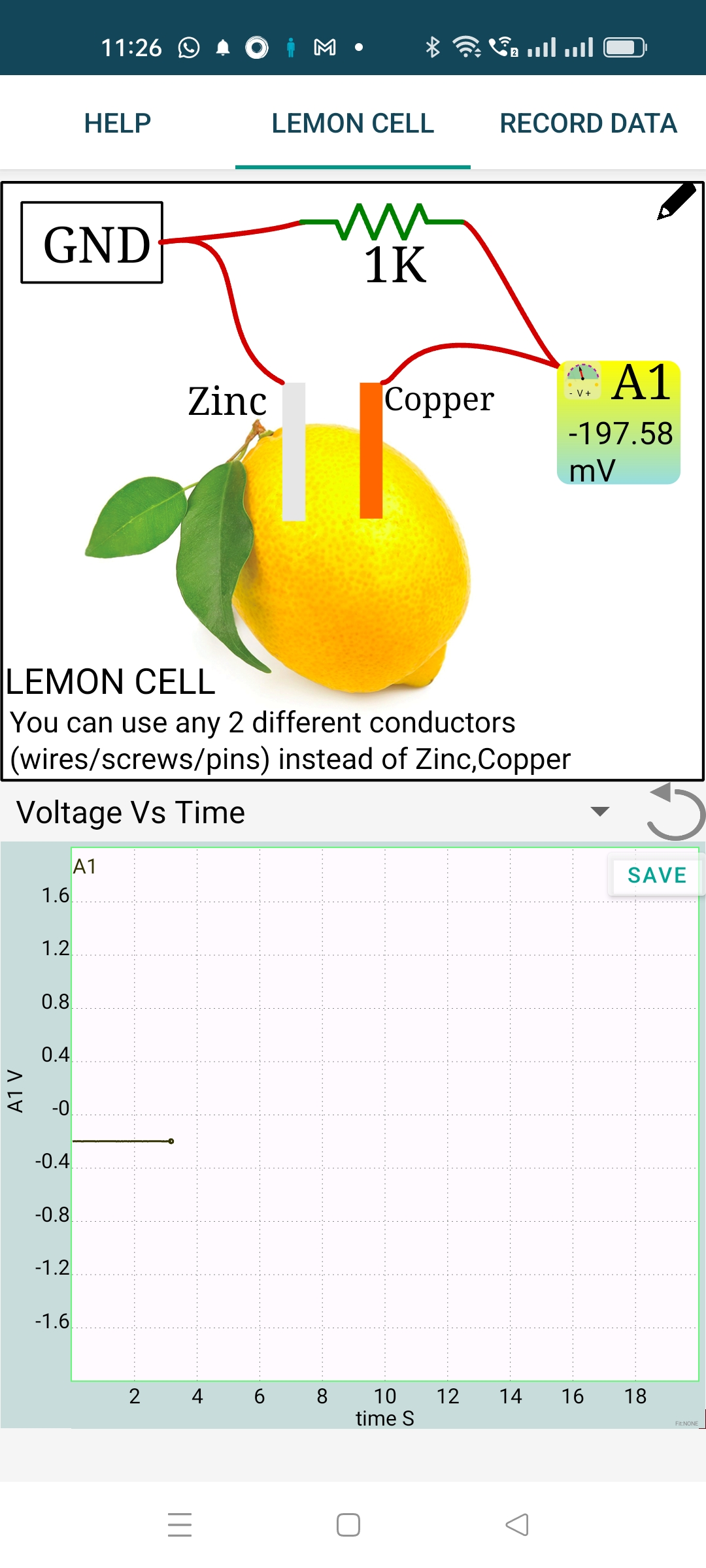# ExpEYES

## Lemon Cell

Electric current is created by separating the opposite charges contained in matter. There are certain chemical reactions that ionize atoms by separating an electron from it. The electrochemical cell utilizes this process, demonstrating the connection between chemistry and electricity. The cell consists of two elecrodes dipped in an electrolyte. Slightly acidic solutions are used as electrolyte. The voltage produced is the difference in the electrode potentials of the two electrodes. The electrode potential depends on the amount of energy released by the chemical reaction where an atom of the electrode leaves two electrons behind and moves to the electrolyte.The cell shown in the figure uses Zinc and Copper electrdes in sulphuric acid, cotaining H+ and $SO_4^{- -}$ ions. An atom of the zinc electrode gives up two electrons and the $Zn^{++}$ ion dissolves in the electrolyte. The electrons travels via the external wire and reaches the Copper electrode where it combines with the H+ ions to release hydrogen gas.

### Source of Energy

During the chemical processes Copper remains intact. Metallic Zinc gets converted into Zinc Sulphate, a process that releases energy. The electrical energy that we are getting is part of the energy spent in making the metallic Zinc. The claims like ‘electrcity from lemon’ or ‘energy from sea water’ are misleading.

### Procedure

• Insert a copper plate and a zinc plate into a lemon. I this experiment we have used a brass screw and a galvanized iron screw. The voltage generated is somewhat less in this case.
• Connect the lemon cell between A1 and GND, as shown in the figure below.

The DC voltage is continuosly measured and plotted. The GUI of the Android version is shown on the left, the measured value is displayed on the A1 icon. In the PC version you need to tick the checkbox A1 (below Measure Voltages) to display the measured DC value.### Internal Resistance of a Cell

What is the difference between a dry cell and our lemon cell. The 1KOhm load connected across the electrodes reduces the measured voltage from 0.9 volts to 0.6 volts, what happened to the remaining 0.3 volts. It is dropped across the internal resistance of the cell. The current through the resistor is given by $I=\frac{V}{R} = \frac{0.6}{1000} = 0.0006 A$. The same amount of current has to flow through the cell also. The resistance of the cell is given by $R=\frac{V}{I} = \frac{0.3}{0.0006} = 500\Omega$. This value depends on the area of the electrode and also it will change with time, due to the bubble formation at the copper electrode.